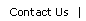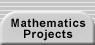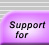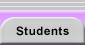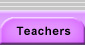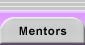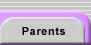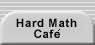# Numbers and Infinity

Students regard numbers as a familiar and well-understood area of mathematics. However, the many types of numbers and representations provide numerous challenging ideas that are important in many research contexts.

## Number Types and Representations

How we represent a set of numbers (using fractions, decimals, radicals, or even different bases) can make them more or less informative. Students may not glean anything from the sequence 1.732…, 2.236…, 2.645…, … but they would certainly notice a pattern in the square roots of 3, 5, and 7. It is important that students seek such alternative representations.

What is ... "How Many?" discusses the integers, rationals, and reals.

What is a number? is a quick introduction to many number types and categories from the reals to perfect numbers to the surreals.

Ask Dr. Math: FAQ: Number Bases answers many questions about representing numbers in different bases and carrying out operations in different bases.

## Numbers to Know About

The Strong Law of Small Numbers: There aren't enough small numbers to meet the many demands made on them. - Richard Guy

Guy’s law is actually concerned with the fact that the numbers with which we are familiar are insufficient for distinguishing between the many patterns that we study. However, the law also highlights the fact that familiar numbers are our tools for most of our work. We should, therefore, be well-versed with them. Students should have a notebook section (or class walls should have posters) that describe and give examples of the following numbers: perfect squares and cubes, prime numbers, triangular numbers, Fibonacci numbers, counting numbers in base 2, and decimal approximations of common fractions, roots, and trigonometric values. As special number types arise during explorations, students can add them to their reference lists.

Download Common Numbers for a sample student reference page.

The Favorite Mathematical Constants page lists a few familiar constants and many other important but less well-known ones that are fun to read about.

Can’t figure out the pattern behind a sequence of numbers? The Online Encyclopedia of Integer Sequences allows you to search for sequences that match numbers that you have found during an investigation. The welcome page provides some background information on the encyclopedia.

## Infinity

The following resources provide background information about, detailed explanations of, and lesson plans on infinity.

Infinity: You can’t get there from here! is a good introductory essay on Cantor’s work on cardinal numbers.

MegaMathematics’ Hotel Infinity provides class activities, stories, and support materials for introducing students (from late elementary onward) to problems involving infinite sets.

What is a number? Discusses countability and the real numbers.

Burger, Edward and Michael Starbird (2000). The heart of mathematics: an invitation to effective thinking. Key College Publishing. Emeryville, California. This book has clear chapters with extension problems on both infinity and the real numbers.

Love, William (1989, April). Infinity: the twilight zone of mathematics. Mathematics Teacher, 284-292. This article is a superb, concise, and accessible introduction to Cantor’s work and his first discoveries about different infinities.

Dauben, Joseph (1983, June). Georg Cantor and the origins of transfinite set theory. Scientific American, 122-131. This article offers a history of Cantor’s life and a technical presentation of his ideas.

Zeno’s Paradox of the Tortoise and Achilles and Limits of the Platonic Realms Interactive Mathematics Encyclopedia discuss problems related to infinite processes.Translations of mathematical formulas for web display were created by tex4ht. © Copyright 2003 Education Development Center, Inc. (EDC)